# Excercise 3.3 Data Handling - NCERT Solutions Class 7

Go back to  'Data Handling'

## Chapter 3 Ex.3.3 Question 1

Use the bar graph (see below figure) to answers the following questions.

(i) Which is the most popular pet?

(ii) How many students have dog as a pet?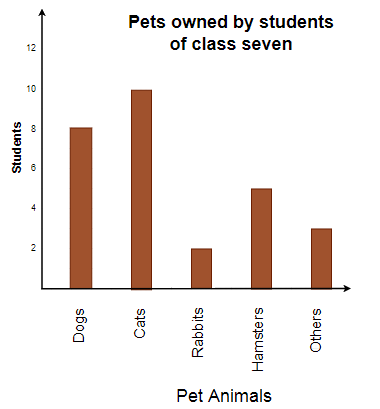### Solution

Steps:

From the given bar graph in figure, we have

(i) Cats are the most popular pet among the students.

(ii) 8 students have dog as a pet animal.

## Chapter 3 Ex.3.3 Question 2

Use the bar graph (see below figure) which shows the no. of books sold by a bookstore during $$5$$ consecutive years and answer the following questions:

(i) About how many books were sold in $$1989, 1990, \text &\,\, 1992 ?$$

(ii) In which year was about $$475$$ books sold? About $$225$$ books sold?

(iii) In which years were fewer than $$250$$ books sold?

(iv) Can you explain how you would estimate the number of books sold in $$1989?$$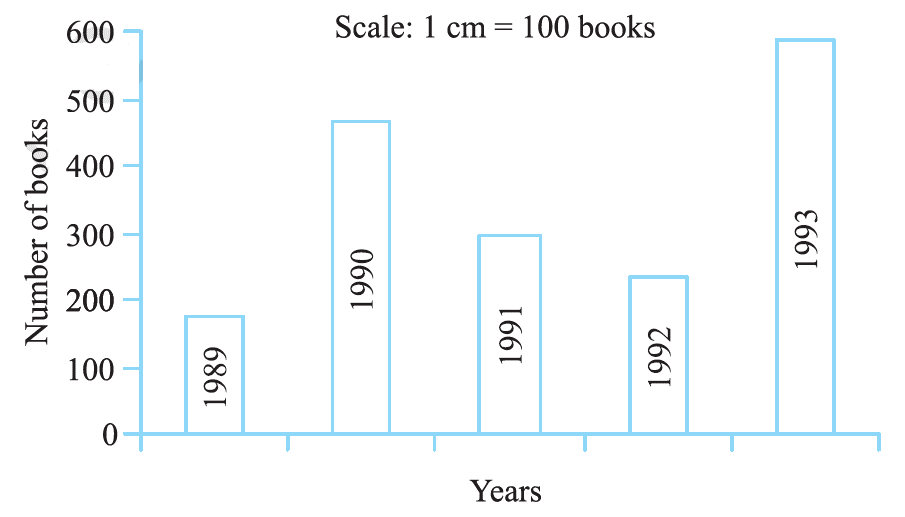### Solution

What is known?

Number of books sold during five consecutive years.

Reasoning:

We can answer all the question by observing the bar graph

Steps:

From the given bar graph, we have

(i) In $$1989 ,180$$ books were sold.

• In $$1990, 475$$ books were sold.
• In $$1992, 225$$ books were sold.

(ii) In $$1990$$ about $$475$$ books were sold and in $$1992$$ about $$225$$ books were sold.

• In $$1989$$ and $$1992$$ fewer than $$250$$ books were sold.
• From the graph we can conclude that $$180$$ books were sold in $$1989.$$

## Chapter 3 Ex.3.3 Question 3

Number of children in six different classes is given below. Represent the data on a bar graph.

 Class Fifth Sixth Seventh Eighth Ninth Tenth Number of Children $$135$$ $$120$$ $$95$$ $$100$$ $$90$$ $$80$$
1. How would you choose a scale?

(i) Which class has the maximum number of children? And minimum?

(ii) Find the ratio of students of class sixth to the students of class eight?

### Solution

What is known?

Number of children in different classes.

Reasoning:

We have to draw the graph by using the data.

Steps: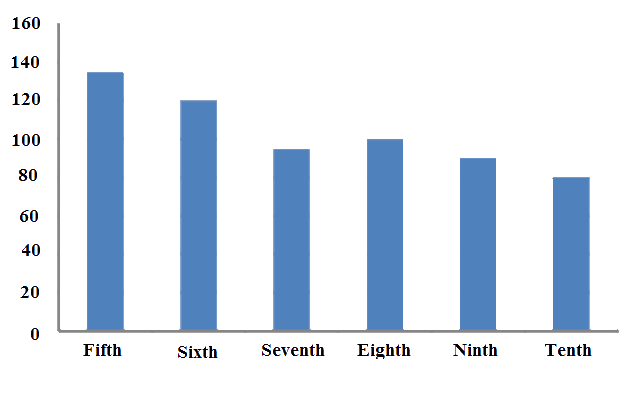a) Scale on y-axis is $$1$$ unit $$= 10$$ children

b)

(i)

• Fifth class has maximum number of children i.e. $$135$$
• Tenth class has the minimum number of children i.e. $$80$$

(ii)

Number of students in class sixth $$=$$ $$120$$

Number of students in class eighth $$=$$ $$100$$

\begin{align} \text{Ratio }&=\frac{\left( \begin{align} & \text{Number of students} \\ & \text{in class sixth} \\ \end{align} \right)}{\left( \begin{align} & \text{Number of students} \\ & \text{in class eighth} \\ \end{align} \right)} \\ & =\frac{120}{100} \\ & =\frac{6}{5} \\ & =6:5 \end{align}

## Chapter 3 Ex.3.3 Question 4

The performance of a student in $$1^{st}$$ term and $$2^{nd}$$ term is given. Draw a double bar graph choosing appropriate scale and answer the following:

 Subject English Hindi Maths Science S. Science $$1^{st}$$ Term (M.M. $$100$$) $$67$$ $$72$$ $$88$$ $$81$$ $$73$$ $$2^{nd}$$ Term (M.M. $$100$$) $$70$$ $$65$$ $$95$$ $$85$$ $$75$$

(i) In which subject, has the child improved his performance the most?

(ii) In which subject improvement is the least?

(iii) Has the performance gone down in any subject?

### Solution

What is known?

Marks obtained in different subject

Reasoning:

We have to draw the graph by using the data

Steps: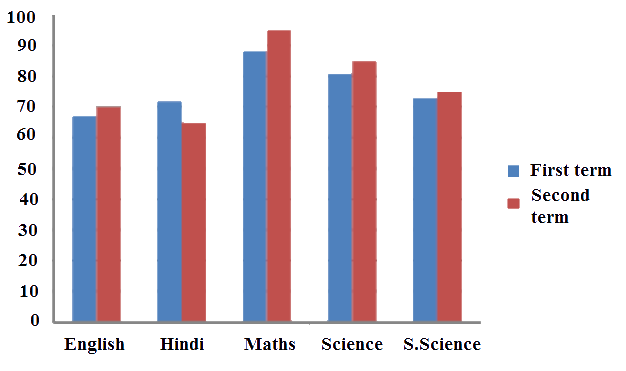Difference between the marks of $$1^{st}$$ and $$2^{nd}$$ term

English $$= 70 \, – 67 = 3$$

Hindi $$= 65 \,– 72 = -7$$ (Decrease in marks)

Math $$= 95\, – 88 = 7$$

Science $$= 85\, – 81= 4$$

Social Science $$= 75\, – 73 =2$$

(i) In Math, the performance of the students improved.

(ii) In Social .science, the performance of the students improved is the least.

(iii)Yes, in Hindi the performance of the students has gone down.

## Chapter 3 Ex.3.3 Question 5

Consider this data collected from a survey of a colony.

 Favourite Sport Cricket Basketball Swimming Hockey Athletics Watching $$1240$$ $$470$$ $$510$$ $$430$$ $$250$$ Participating $$620$$ $$320$$ $$320$$ $$250$$ $$105$$

(i) Draw a double bar graph choosing an appropriate scale.
What do you infer from the bar graph?

(ii) Which sport is most popular?

(iii) Which is more preferred, watching or participating in the sports?

### Solution

Reasoning:

We have to draw the graph by using the data

Steps: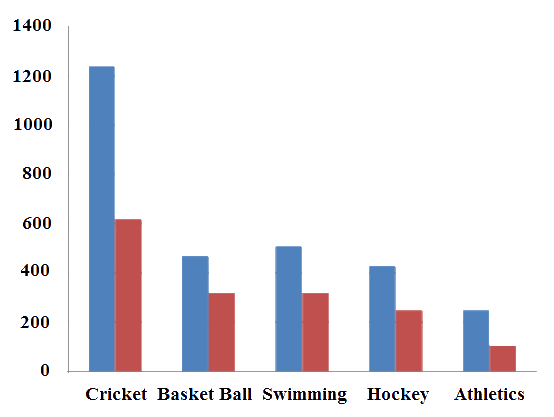(i) This bar graph shows the number of persons who are watching and participating in their favorite sports.

(ii) Cricket is the most popular sport.

(iii) Watching different sports is more preferred than participating in the sports.

## Chapter 3 Ex.3.3 Question 6

Take the data giving the maximum and minimum temperatures of various cities given in the beginning of this chapter (in the table below). Draw a double bar graph using the data and answer the following.

(i) Which city has the highest difference in the minimum and maximum temperature on the given date?

(ii) Which is the hottest city and which is the coldest city?

(iii) Name two cities where maximum temperature of one was less than the minimum temperature of the other.

(iv) Name the city which has the least difference between its minimum and maximum temperature?

### Solution

Steps: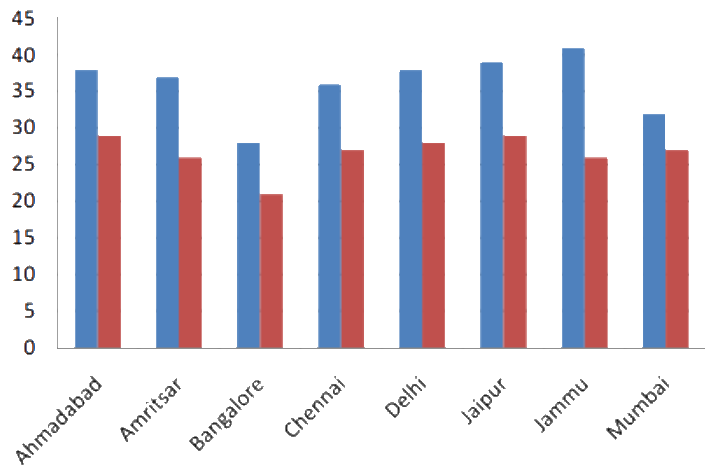Temperature of cities as on $$20.06.2006$$

 City Maximum Minimum Ahmedabad $$38^\circ \rm C$$ $$29 ^\circ \rm C$$ Amritsar $$37 ^\circ \rm C$$ $$26 ^\circ \rm C$$ Bangalore $$28 ^\circ \rm C$$ $$21 ^\circ \rm C$$ Chennai $$36 ^\circ \rm C$$ $$27 ^\circ \rm C$$ Delhi $$38^\circ \rm C$$ $$28 ^\circ \rm C$$ Jaipur $$39 ^\circ \rm C$$ $$29 ^\circ \rm C$$ Jammu $$41 ^\circ \rm C$$ $$26 ^\circ \rm C$$ Mumbai $$32 ^\circ \rm C$$ $$27 ^\circ \rm C$$

Difference between the maximum and minimum temperature on $$20.06.2006$$

Ahmedabad $$= (38^\circ \rm C - 29^\circ \rm C) = 9^\circ \rm C$$

Amritsar $$=$$ ($$37 ^\circ \rm C$$ $$-$$ $$26^\circ \rm C$$) $$=$$ $$9 ^\circ \rm C$$

Bangalore $$=$$ ($$28^\circ \rm C$$ $$-$$ $$21^\circ \rm C$$) $$=$$ $$7 ^\circ \rm C$$

Chennai $$=$$ ($$36^\circ \rm C$$ $$-$$ $$27^\circ \rm C$$) $$=$$ $$9 ^\circ \rm C$$

Delhi $$=$$ ($$38 ^\circ \rm C$$ $$-$$ $$28^\circ \rm C$$) $$=$$ $$10 ^\circ \rm C$$

Jaipur $$=$$ ($$39 ^\circ \rm C$$ $$-$$ $$29 ^\circ \rm C$$) $$=$$ $$10 ^\circ \rm C$$

Jammu $$=$$ ($$41 ^\circ \rm C$$ $$-$$ $$26^\circ \rm C$$) $$=$$ $$15 ^\circ \rm C$$

Mumbai $$=$$ ($$32 ^\circ \rm C$$ $$-$$ $$27^\circ \rm C$$) $$=$$ $$5^\circ \rm C$$

(i)  As, it is clear from the above calculation, Jammu has the largest difference in the minimum and maximum temperature on the given data i.e. $$=$$ $$15 ^\circ \rm C$$.

(ii) Jammu is the hottest city with $$41^\circ \rm C$$ and Bangalore is the coldest city with $$21^\circ \rm C$$.

Bangalore and Jaipur or Bangalore and Ahmedabad are the two cities where maximum temperature of one was less than the minimum temperature of other.

Mumbai has the least difference between its minimum and maximum temperature.
Maximum temperature $$= 32^\circ \rm C$$
Minimum temperature $$=$$ $$27^\circ \rm C$$
∴ Difference $$=$$ ($$32 ^\circ \rm C$$ $$-$$ $$27^\circ \rm c$$) $$=$$ $$5^\circ \rm C$$

Related Sections
Related Sections
Instant doubt clearing with Cuemath Advanced Math Program